# How to Calculate and Solve for the Width, Height, Length and Volume of a Rectangular Prism | Nickzom Calculator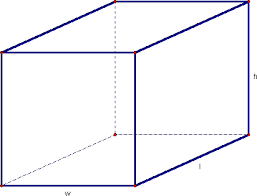The image above is a rectangular prism.

To compute the volume of a rectangular prism requires three essential parameters which are the length, width and height of the rectangular prism.

V = lwh

Where;
V = Volume of the rectangular prism
l = Length of the rectangular prism
w = Width of the rectangular prism
h = Height of the rectangular prism

Let’s solve an example;
Find the volume of a rectangular prism with a length of 18 cm, a width of 12 cm and a height of 21 cm.

This implies that;
l = Length of the rectangular prism = 18 cm
w = Width of the rectangular prism = 12 cm
h = Height of the rectangular prism = 21 cm

V = lwh
V = 18 x 12 x 21
V = 4536

Therefore, the volume of the rectangular prism is 4536 cm3.

Calculating the Length of a Rectangular Prism using the Volume, Width and Height of the Rectangular Prism.

l = V / wh

Where;
l = Length of the rectangular prism
V = Volume of the rectangular prism
w = Width of the rectangular prism
h = Height of the rectangular prism

Let’s solve an example;
Find the length of a rectangular prism with a width of 19 cm, a height of 24 cm and a Volume of 250 cm3.

This implies that;
w = Width of the rectangular prism = 19 cm
h = Height of the rectangular prism = 24 cm
V = Volume of the rectangular prism = 250 cm3

l = V / wh
l = 250 / 19 x 24
l = 250 / 456
l = 0.55

Therefore, the length of the rectangular prism is 0.55 cm.

Calculating the Width of the Rectangular Prism using the Height, the Volume and the Length of the Rectangular Prism.

w = V / lh

Where;
w = Width of the rectangular prism
V = Volume of the rectangular prism
l = Length of the rectangular prism
h = height of the rectangular prism

Let’s solve an example;
Given that the height of a rectangular prism is 30 cm, the Volume is 320 cm3 and the length is 15 cm. Find the width of the rectangular prism?

This implies that;
h = Height of the rectangular prism = 30 cm
V = Volume of the rectangular prism = 320 cm3
l = Length of the rectangular prism = 15 cm

w = V / lh
w = 320 / 15 x 30
w = 320 / 450
w = 0.71

Therefore, the width of the rectangular prism is 0.71 cm.

Calculating the Height of the Rectangular Prism using the Width, the Volume and the Length of the Rectangular Prism.

h = V / lw

Where;
w = Width of the rectangular prism
V = Volume of the rectangular prism
l = Length of the rectangular prism
h = height of the rectangular prism

Let’s solve an example;
Given that the width of a rectangular prism is 25 cm, the Volume is 220 cm3 and the length is 19 cm. Find the height of the rectangular prism?

This implies that;
w = Width of the rectangular prism = 25 cm
V = Volume of the rectangular prism = 220 cm3
l = Length of the rectangular prism = 19 cm

h = V / lw
h = 220 / 19 x 25
h = 220 / 475
h = 0.46

Therefore, the height of the rectangular prism is 0.46 cm.

Nickzom Calculator – The Calculator Encyclopedia is capable of calculating the volume of a rectangular prism.

To get the answer and workings of the volume of a rectangular prism using the Nickzom Calculator – The Calculator Encyclopedia. First, you need to obtain the app.

You can get this app via any of these means:

To get access to the professional version via web, you need to register and subscribe for NGN 1,500 per annum to have utter access to all functionalities.
You can also try the demo version via https://www.nickzom.org/calculator

Once, you have obtained the calculator encyclopedia app, proceed to the Calculator Map, then click on Mensuration under the Mathematics section.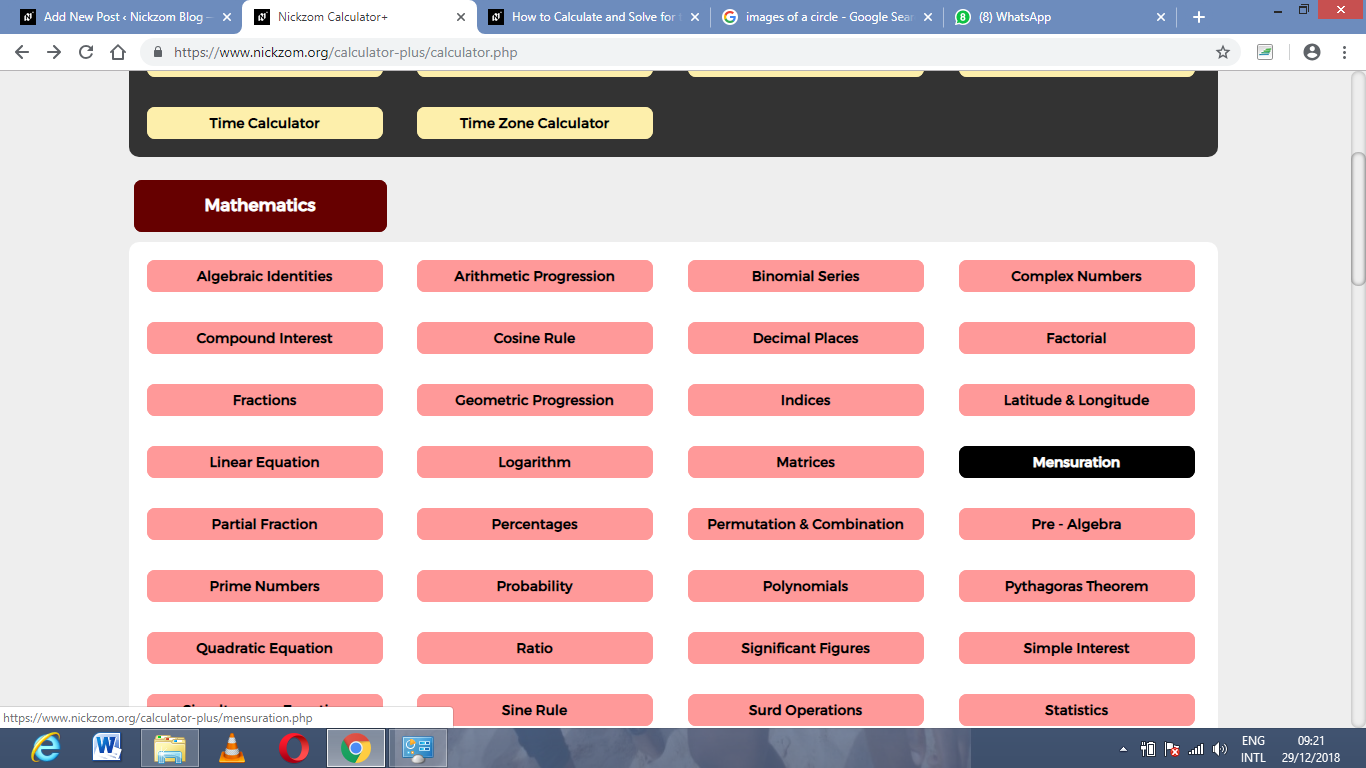Now, click on Volume of a rectangular prism under MensurationThe screenshot below displays the page or activity to enter your values, to get the answer for the volume of a rectangular prism according to the respective parameters which are the length, the width and the height of the rectangular prism.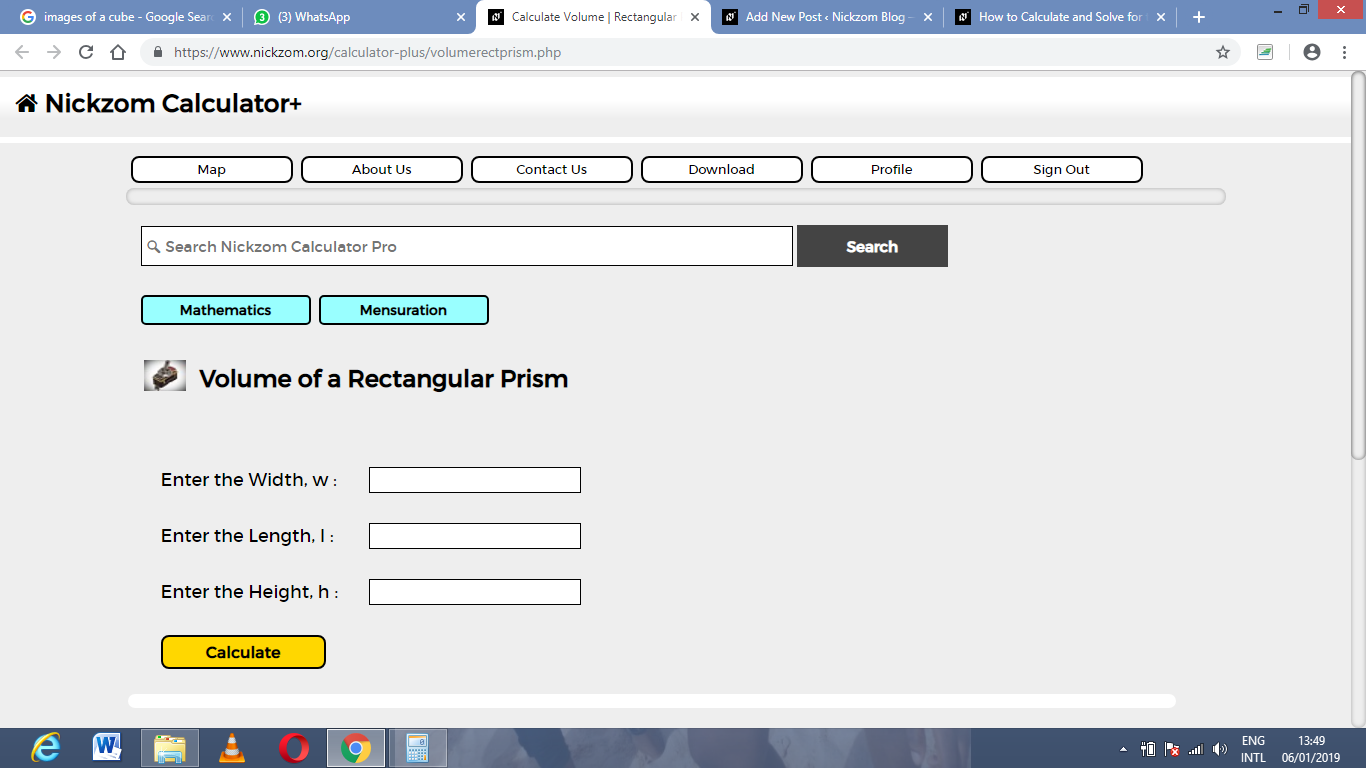Now, enter the values appropriately and accordingly for the parameters as required by the example above where the width is 12 cm, the length is 18 cm and the height is 21 cm.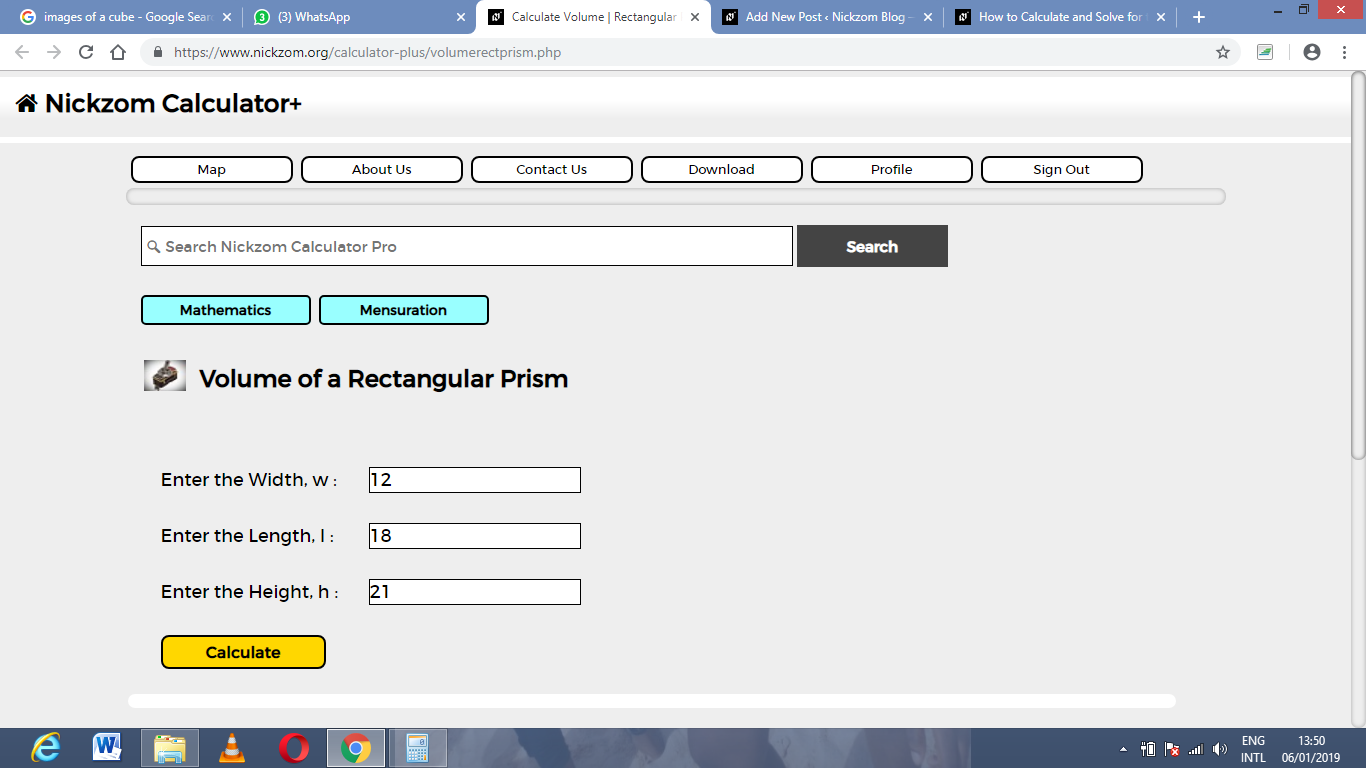Finally, Click on Calculate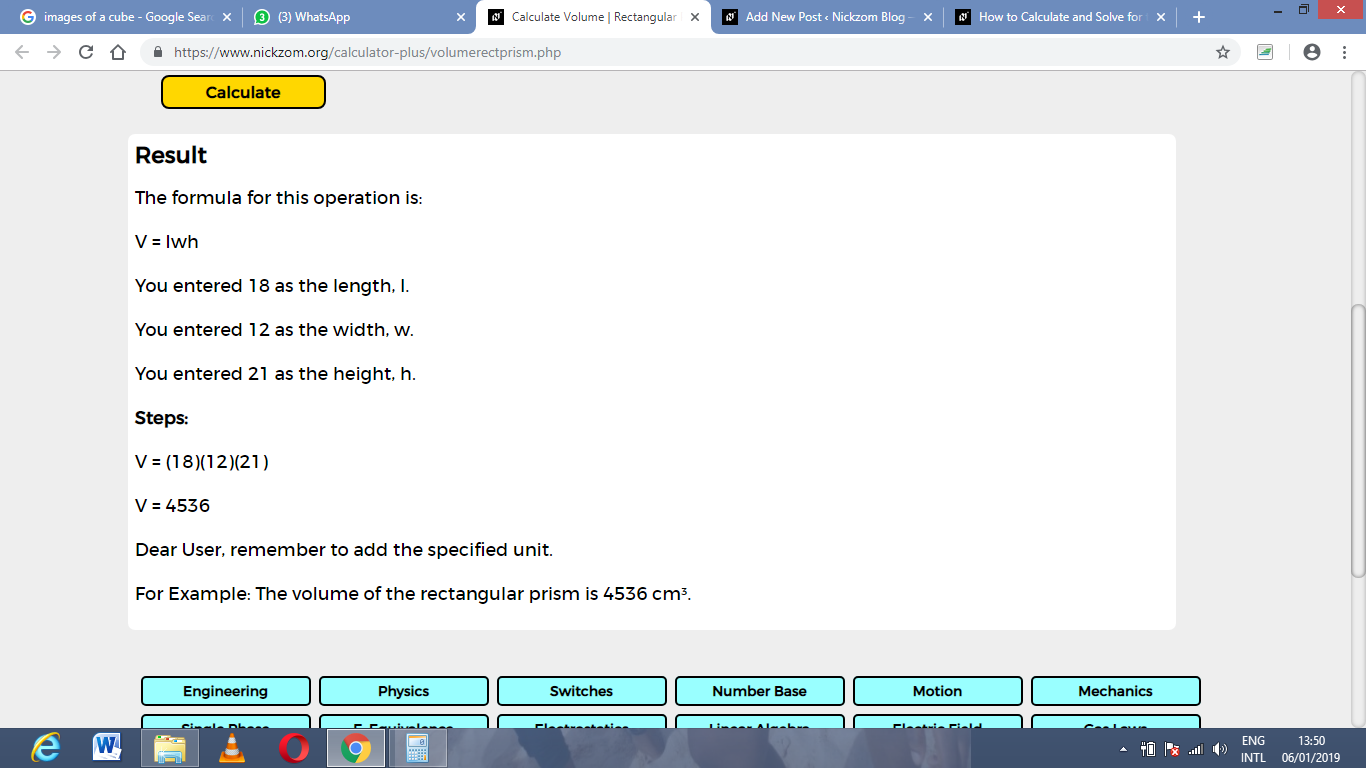As you can see from the screenshot above, Nickzom Calculator – The Calculator Encyclopedia solves for the volume of a rectangular prism and presents the formula, workings and steps too.